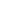Making (near) Optimal Choices for the Design of Block Ciphers ( Baptiste Lambin , Horst Görtz Institute for IT-Security, Ruhr-University Bochum)

One of the many ways to build encryption algorithm in symmetric cryptography is to design what's called a block cipher : a family of permutations indexed by a key, such that choosing a key select one permutation, but it's hard to find the key for a given permutation. The encryption of a given plaintext is then simply its image through the chosen permutation. When designing block ciphers, we need to make decisions on which specific components to use (e.g. S-boxes, linear layer etc.). These decisions are made by taking into account the security of the resulting block cipher, but also the underlying cost in term of performances. In this talk, I will present two different works aiming at finding optimal components w.r.t a given criteria, while also trying to keep a very cheap implementation cost. I will show how the use of automatic tools (either already existing or specifically designed) can help us to make (near) optimal decisions while the initial search space is very large. After an general introduction to present the context in more details, I will first focus a block cipher construction called Generalized Feistel Networks, which is a very efficient construction in general. The idea of this construction is to split the plaintext into an even number of block, apply a Feistel transformation in parallel to those blocks, and then permute them. In a recent paper accepted at FSE 2020, we showed how to choose this permutation to be optimal w.r.t a certain criterion (called "diffusion round"), which also allowed us to solve a 10-year old problem. The second part of my talk will be based on a paper accepted at SAC 2018, which aims at replacing the key-schedule of AES by a more efficient one (namely a permutation). AES is the current symmetric encryption standard, and its key-schedule (i.e. the algorithm allowing to transform the master key into several round keys) is quite intricate and costly. We thus studied what would happen if we replace this key-schedule by a permutation, and especially we show that we can get better security arguments even though we have a much simpler key-schedule.

130 vues
Mercredi 26 Février 2020
Tag(s) : Confirance numérique, Cryptographie
Intégrer :
Vidéos suggérées